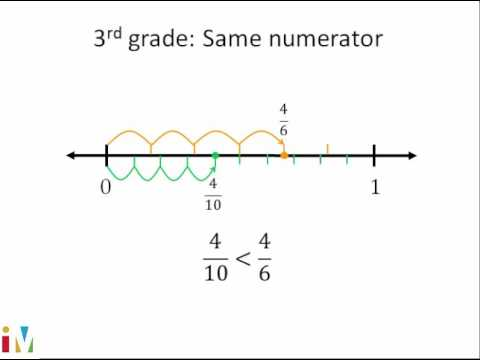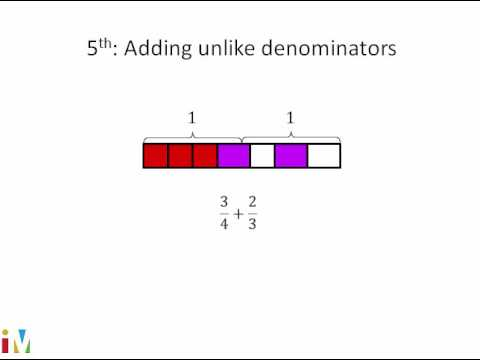# Visually adding fractions: 5/6 + 1/4 (Full video)

Description: Sal adds 5/6 + 1/4 using a fraction model for help. Notice I have this whole whole, I guess you could say, broken up into one, two, three, four, five, six sections, and we've shaded in five of them, so this is 5/6, and then down here, we have another whole, and we have one out of the four equal sections shaded in so this is 1/4, and I want to add them, and I encourage you at any point, pause the video, and see if you could figure it out on your own. Six is clearly divisible by six, but it's not perfectly divisible by four, so now, let's multiply by two, so then we get to 12. I'm essentially multiplying each of these sections by, or, I'm essentially multiplying the number of sections I have by two, or I'm taking each of these existing sections and I'm turning them into two sections, so let's do that.

### Other videos you might be interested in### Comparing Fractions

#### Illustrative Mathematics